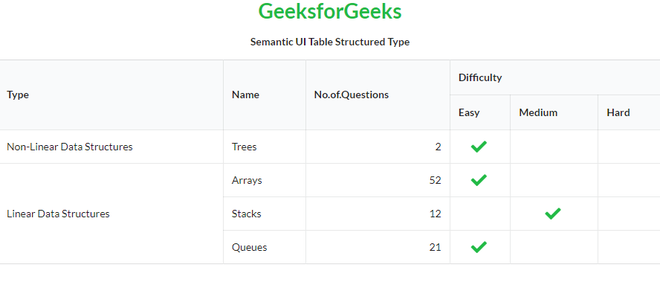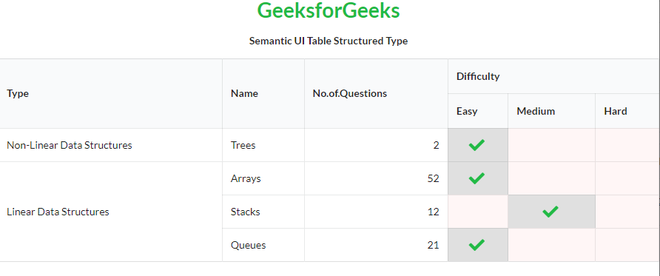GFG App
Open AppBrowser
Continue

# Semantic-UI Table Structured Type

Semantic UI is an open-source framework that uses CSS and jQuery to build great user interfaces. It is the same as a bootstrap for use and has great different elements to use to make your website look more amazing. It uses a class to add CSS to the elements.

Tables are an easy way to organize a lot of data. A table is an arrangement of data in rows and columns, or possibly in a more complex structure. Tables are widely used in communication, research, and data analysis. Tables are useful for various tasks such as presenting text information and numerical data. It can be used to compare two or more items in the tabular form layout. Tables are used to create databases. An HTML table and a Semantic UI table both are the same structurally.

In the case of a Structured Type of table, it is formatted to display complex structured data.

Semantic UI Table Structured Type Class:

• structured: This class defines that the type of table is structured.

Syntax:

```<table class="ui structured table">
<tr>
<td></td>
...
</tr>
...
</table>```

Example 1: This is a basic example illustrating Table Structured Type made using Semantic UI.

## HTML

 ` ` `<``html``> ` ` `  `<``head``> ` `    ``<``title``>Semantic UI Table Structured Type ` `    ``<``link` `href``= ` `"https://cdnjs.cloudflare.com/ajax/libs/semantic-ui/2.4.1/semantic.min.css"` `        ``rel``=``"stylesheet"` `/> ` `    ``<``script` `src``=``"https://code.jquery.com/jquery-3.1.1.min.js"` `        ``integrity``=``"sha256-hVVnYaiADRTO2PzUGmuLJr8BLUSjGIZsDYGmIJLv2b8="`  `        ``crossorigin``=``"anonymous"``>  ` `    `` ` `    ``<``script` `src``= ` `"https://cdnjs.cloudflare.com/ajax/libs/semantic-ui/2.4.1/semantic.min.js"``> ` `    `` ` ` ` ` `  `<``body``> ` `    ``<``center``> ` `        ``<``h1` `class``=``"ui green header"``>GeeksforGeeks ` `        ``<``strong``>Semantic UI Table Structured Type ` `    `` ` `    ``<``table` `class``=``"ui celled  structured table"``> ` `        ``<``thead``> ` `            ``<``tr``> ` `                ``<``th` `rowspan``=``"2"``>Type ` `                ``<``th` `rowspan``=``"2"``>Name ` `                ``<``th` `rowspan``=``"2"``>No.of.Questions ` `                ``<``th` `colspan``=``"3"``>Difficulty ` `            `` ` `            ``<``tr``> ` `                ``<``th``>Easy ` `                ``<``th``>Medium ` `                ``<``th``>Hard ` `            `` ` `        `` ` `        ``<``tbody``> ` `            ``<``tr``> ` `                ``<``td``>Non-Linear Data Structures ` `                ``<``td``>Trees ` `                ``<``td` `class``=``"right aligned"``>2 ` `                ``<``td` `class``=``"center aligned"``> ` `                    ``<``i` `class``=``"large green checkmark icon"``> ` `                `` ` `                ``<``td``> ` `                ``<``td``> ` `            `` ` `            ``<``tr``> ` `                ``<``td` `rowspan``=``"3"``>Linear Data Structures ` `                ``<``td``>Arrays ` `                ``<``td` `class``=``"right aligned"``>52 ` `                ``<``td` `class``=``"center aligned"``> ` `                    ``<``i` `class``=``"large green checkmark icon"``> ` `                `` ` `                ``<``td``> ` `                ``<``td``> ` `            `` ` `            ``<``tr``> ` `                ``<``td``>Stacks ` `                ``<``td` `class``=``"right aligned"``>12 ` `                ``<``td``> ` `                ``<``td` `class``=``"center aligned"``> ` `                    ``<``i` `class``=``"large green checkmark icon"``> ` `                `` ` `                ``<``td``> ` `            `` ` `            ``<``tr``> ` `                ``<``td``>Queues ` `                ``<``td` `class``=``"right aligned"``>21 ` `                ``<``td` `class``=``"center aligned"``> ` `                    ``<``i` `class``=``"large green checkmark icon"``> ` `                `` ` `                ``<``td``> ` `                ``<``td``> ` `            `` ` `        `` ` `    `` ` ` ` ` `  ``

Output:Example 2: This is a basic example illustrating Table Structured Type with different States made using Semantic UI.

## HTML

 ` ` `<``html``> ` ` `  `<``head``> ` `    ``<``title``>Semantic UI Table Structured Type ` `    ``<``link` `href``= ` `"https://cdnjs.cloudflare.com/ajax/libs/semantic-ui/2.4.1/semantic.min.css"` `        ``rel``=``"stylesheet"` `/> ` `    ``<``script` `src``=``"https://code.jquery.com/jquery-3.1.1.min.js"` `        ``integrity``=``"sha256-hVVnYaiADRTO2PzUGmuLJr8BLUSjGIZsDYGmIJLv2b8="`  `        ``crossorigin``=``"anonymous"``>  ` `    `` ` `    ``<``script` `src``= ` `"https://cdnjs.cloudflare.com/ajax/libs/semantic-ui/2.4.1/semantic.min.js"``> ` `    `` ` ` ` ` `  `<``body``> ` `    ``<``center``> ` `        ``<``h1` `class``=``"ui green header"``>GeeksforGeeks ` `        ``<``strong``>Semantic UI Table Structured Type ` `    `` ` `    ``<``table` `class``=``"ui celled  structured table"``> ` `        ``<``thead``> ` `            ``<``tr``> ` `                ``<``th` `rowspan``=``"2"``>Type ` `                ``<``th` `rowspan``=``"2"``>Name ` `                ``<``th` `rowspan``=``"2"``>No.of.Questions ` `                ``<``th` `colspan``=``"3"``>Difficulty ` `            `` ` `            ``<``tr``> ` `                ``<``th``>Easy ` `                ``<``th``>Medium ` `                ``<``th``>Hard ` `            `` ` `        `` ` `        ``<``tbody``> ` `            ``<``tr``> ` `                ``<``td``>Non-Linear Data Structures ` `                ``<``td``>Trees ` `                ``<``td` `class``=``"right aligned"``>2 ` `                ``<``td` `class``=``"center aligned active"``> ` `                    ``<``i` `class``=``"large green checkmark icon"``> ` `                `` ` `                ``<``td` `class``=``"error"``> ` `                ``<``td` `class``=``"error"``> ` `            `` ` `            ``<``tr``> ` `                ``<``td` `rowspan``=``"3"``>Linear Data Structures ` `                ``<``td``>Arrays ` `                ``<``td` `class``=``"right aligned"``>52 ` `                ``<``td` `class``=``"center aligned active"``> ` `                    ``<``i` `class``=``"large green checkmark icon"``> ` `                `` ` `                ``<``td` `class``=``"error"``> ` `                ``<``td` `class``=``"error"``> ` `            `` ` `            ``<``tr``> ` `                ``<``td``>Stacks ` `                ``<``td` `class``=``"right aligned"``>12 ` `                ``<``td` `class``=``"error"``> ` `                ``<``td` `class``=``"center aligned active"``> ` `                    ``<``i` `class``=``"large green checkmark icon"``> ` `                `` ` `                ``<``td` `class``=``"error"``> ` `            `` ` `            ``<``tr``> ` `                ``<``td``>Queues ` `                ``<``td` `class``=``"right aligned"``>21 ` `                ``<``td` `class``=``"center aligned active"``> ` `                    ``<``i` `class``=``"large green checkmark icon"``> ` `                `` ` `                ``<``td` `class``=``"error"``> ` `                ``<``td` `class``=``"error"``> ` `            `` ` `        `` ` `    `` ` ` ` ` `  ``

Output:My Personal Notes arrow_drop_up